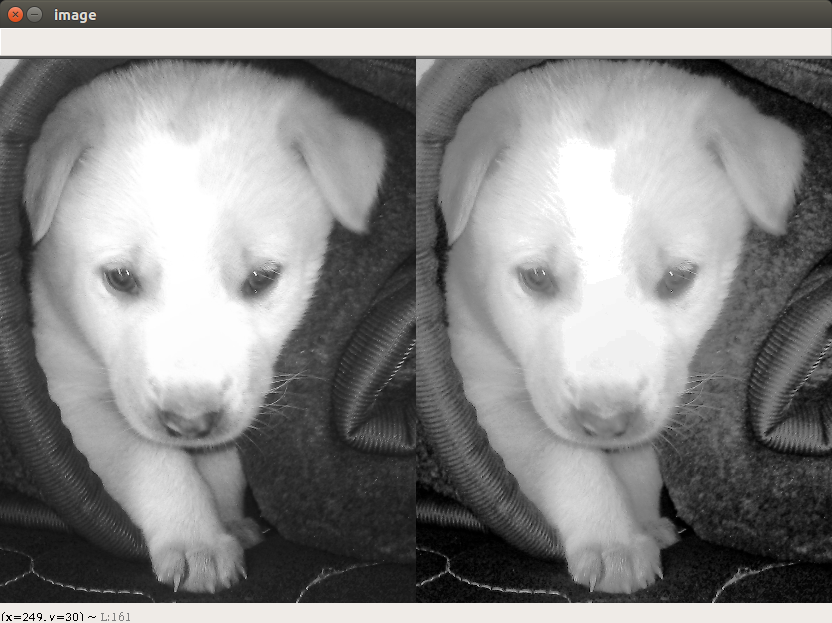# Histograms Equalization in OpenCV

• Last Updated : 22 Feb, 2018

Prerequisite : Analyze-image-using-histogram

Histogram equalization is a method in image processing of contrast adjustment using the image’s histogram.

This method usually increases the global contrast of many images, especially when the usable data of the image is represented by close contrast values. Through this adjustment, the intensities can be better distributed on the histogram. This allows for areas of lower local contrast to gain a higher contrast. Histogram equalization accomplishes this by effectively spreading out the most frequent intensity values. The method is useful in images with backgrounds and foregrounds that are both bright or both dark.

OpenCV has a function to do this, cv2.equalizeHist(). Its input is just grayscale image and output is our histogram equalized image.

Input Image :## Recommended: Please try your approach on {IDE} first, before moving on to the solution.

Below is Python3 code implementing Histogram Equalization :

 `# import Opencv ` `import` `cv2 ` ` `  `# import Numpy ` `import` `numpy as np ` ` `  `# read a image using imread ` `img ``=` `cv2.imread(\``'F:\\do_nawab.png\'``, ``0``) ` ` `  `# creating a Histograms Equalization ` `# of a image using cv2.equalizeHist() ` `equ ``=` `cv2.equalizeHist(img) ` ` `  `# stacking images side-by-side ` `res ``=` `np.hstack((img, equ)) ` ` `  `# show image input vs output ` `cv2.imshow(\``'image\'``, res) ` ` `  `cv2.waitKey(``0``) ` `cv2.destroyAllWindows() `

Output :

``````
My Personal Notes arrow_drop_up
Recommended Articles
Page :